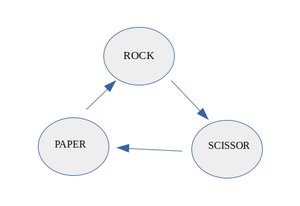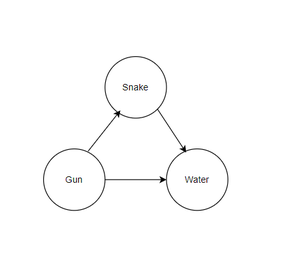Check whether the structure of given Graph is same as the game of Rock-Paper-Scissor

• Last Updated : 15 Jul, 2021

Given an array arr[] of N pairs of strings representing a directed graph, where each pair of the strings represents an edge between the nodes represented by the pair of strings, the task is to check whether the structure of the given directed graph is the same as that of the game of Rock-Paper-Scissor. If found true, then print “Yes“. Otherwise, print “No“.

Note:

In the real game of Rock-Paper-Scissor, the rock dominates over the scissor, scissor dominates over the paper and paper dominates over the rock.The game can be modelled using a directed graph as below, in which the directed edge (a, b) shows that a dominates over b.Examples:

Input: arr[] = {{“Snake”, “Water”}, {“Water”, “Gun”}, {“Gun”, “Snake”}}
Output: Yes
Explanation:Therefore, from the above image it can be seen that the given graph structure is similar to Rock-Paper-Scissor.

Input: arr[]={{“Snake”, “Water”}, {“Water”, “Gun”}, {“Gun”, “Gun”}}
Output: No

Approach: The problem can be solved by using the indegree and outdegree of the graphs based on the observation that in the directed graph Rock-Paper-Scissor there are only three nodes and indegree and outdegree of each node is one. Follow the steps below to solve the problem.

• Initialize two maps of {strings, int} say inDegree and outDegree, to store the indegree and outdegree of each node in these maps.
• Initialize a set of strings say st and insert all the nodes i.e. strings in this set.
• Check if the size of any map is not equal to 3 or the size of the set st of nodes is not equal to 3, then print “No“.
• Iterate over both the maps and check if the second value of any entry map is not 1, then, print “No” and break.
• Finally, if none of the above cases satisfy, then print “Yes“.

Below is the implementation of the above approach:

C++

 // C++ program for the above approach #include using namespace std;   // Function to check if the given directed // graph has same structure to Rock-Paper-Scissor string similarGame(vector > arr) {       // Stores indegree of each node     unordered_map indegree;       // Stores outdegree of each node     unordered_map outdegree;       // Stores all nodes     set st;       // Traverse through the edges     for (int i = 0; i < arr.size(); i++) {           // Check presence of self loop         if (arr[i] == arr[i])             return "No";           // Insert the node arr[i] and arr[i]         // into the set         st.insert(arr[i]);         st.insert(arr[i]);           // Increment the outdegree and indegree of         // nodes arr[i] and arr[i]           outdegree[arr[i]]++;         indegree[arr[i]]++;     }       // Check base conditions     if (outdegree.size() != 3 || indegree.size() != 3         || st.size() != 3)         return "No";       // Traverse the array outdegree     for (auto it : outdegree) {         if (it.second != 1)             return "No";     }       // Traverse the array indegree     for (auto it : indegree) {         if (it.second != 1)             return "No";     }       // Return     return "Yes"; }   // Driver Code int main() {       // Given Input     vector > arr = { { "Snake", "Water" },                                     { "Water", "Gun" },                                     { "Gun", "Snake" } };       // Function Call     cout << similarGame(arr);       return 0; }

Python3

 # Python 3 program for the above approach   # Function to check if the given directed # graph has same structure to Rock-Paper-Scissor def similarGame(arr):     # Stores indegree of each node     indegree = {}       # Stores outdegree of each node     outdegree = {}       # Stores all nodes     st = set()       # Traverse through the edges     for i in range(len(arr)):         # Check presence of self loop         if (arr[i] == arr[i]):             return "No"           # Insert the node arr[i] and arr[i]         # into the set         st.add(arr[i])         st.add(arr[i])           # Increment the outdegree and indegree of         # nodes arr[i] and arr[i]         if arr[i] in outdegree:             outdegree[arr[i]] += 1         else:             outdegree[arr[i]] = 0         if arr[i] in indegree:             indegree[arr[i]] += 1         else:             indegree[arr[i]] = 0       # Check base conditions     if (len(outdegree) != 3 and len(indegree) != 3 and len(st) != 3):         return "No";       # Traverse the array outdegree     for key,value in outdegree.items():         if (value == 1):             return "No"       # Traverse the array indegree     for key,value in indegree.items():         if (value == 1):             return "No"       # Return     return "Yes"   # Driver Code if __name__ == '__main__':     # Given Input     arr = [["Snake", "Water"],["Water", "Gun"],["Gun", "Snake"]]       # Function Call     print(similarGame(arr))           # This code is contributed by SURENDRA_GANGWAR.

Output

Yes

Time Complexity: O(N)
Auxiliary Space: O(N)

My Personal Notes arrow_drop_up
Recommended Articles
Page :